# نتائج البحث (5)

View
Selected filters:
• Calculus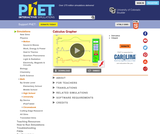Conditions of Use:
No Strings Attached
Rating

Draw a graph of any function and see graphs of its derivative and integral. Don't forget to use the magnify/demagnify controls on the y-axis to adjust the scale.

Subject:
Calculus
Material Type:
Simulation
Provider:
University of Colorado Boulder
Provider Set:
PhET Interactive Simulations
Author:
Michael Dubson
Trish Loeblein
Date Added:
08/31/2009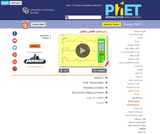Conditions of Use:
قراءة النص المطبوع بخط رفيع
Rating

ارسم الرسم البياني لأي دالة وشاهد الرسوم البيانية لمشتقاتها و أجزائها التكاملية. لا تنس أن تقوم باستخدام ضوابط التكبير/التصغير على محور y لضبط مستوى الحجم.

الموضوع:
علم الحساب
نوع المادة:
محاكاة
Provider:
University of Colorado Boulder
Provider Set:
المحاكاة التفاعلية لموقع PhET
المؤلف:
Michael Dubson
Patricia Loblein
Date Added:
02/02/2013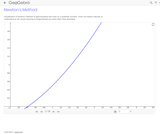Conditions of Use:
Remix and Share
Rating

A visual demo of Newton's Method for pre-calculus students.

Subject:
Calculus
Geometry
Material Type:
Simulation
Provider:
GeoGebra
Provider Set:
GeoGebraTube
Date Added:
04/26/2012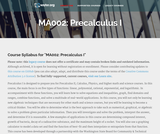Conditions of Use:
قراءة النص المطبوع بخط رفيع
Rating

Precalculus I is designed to prepare you for Precalculus II, Calculus, Physics, and higher math and science courses. In this course, the main focus is on five types of functions: linear, polynomial, rational, exponential, and logarithmic. In accompaniment with these functions, you will learn how to solve equations and inequalities, graph, find domains and ranges, combine functions, and solve a multitude of real-world applications.

الموضوع:
علم الحساب
نوع المادة:
المقرر التعليمي الكامل
Provider:
The Saylor Foundation
Date Added:
08/28/2013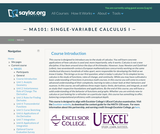Conditions of Use:
قراءة النص المطبوع بخط رفيع
Rating

This course is designed to introduce the student to the study of Calculus through concrete applications. Upon successful completion of this course, students will be able to: Define and identify functions; Define and identify the domain, range, and graph of a function; Define and identify one-to-one, onto, and linear functions; Analyze and graph transformations of functions, such as shifts and dilations, and compositions of functions; Characterize, compute, and graph inverse functions; Graph and describe exponential and logarithmic functions; Define and calculate limits and one-sided limits; Identify vertical asymptotes; Define continuity and determine whether a function is continuous; State and apply the Intermediate Value Theorem; State the Squeeze Theorem and use it to calculate limits; Calculate limits at infinity and identify horizontal asymptotes; Calculate limits of rational and radical functions; State the epsilon-delta definition of a limit and use it in simple situations to show a limit exists; Draw a diagram to explain the tangent-line problem; State several different versions of the limit definition of the derivative, and use multiple notations for the derivative; Understand the derivative as a rate of change, and give some examples of its application, such as velocity; Calculate simple derivatives using the limit definition; Use the power, product, quotient, and chain rules to calculate derivatives; Use implicit differentiation to find derivatives; Find derivatives of inverse functions; Find derivatives of trigonometric, exponential, logarithmic, and inverse trigonometric functions; Solve problems involving rectilinear motion using derivatives; Solve problems involving related rates; Define local and absolute extrema; Use critical points to find local extrema; Use the first and second derivative tests to find intervals of increase and decrease and to find information about concavity and inflection points; Sketch functions using information from the first and second derivative tests; Use the first and second derivative tests to solve optimization (maximum/minimum value) problems; State and apply Rolle's Theorem and the Mean Value Theorem; Explain the meaning of linear approximations and differentials with a sketch; Use linear approximation to solve problems in applications; State and apply L'Hopital's Rule for indeterminate forms; Explain Newton's method using an illustration; Execute several steps of Newton's method and use it to approximate solutions to a root-finding problem; Define antiderivatives and the indefinite integral; State the properties of the indefinite integral; Relate the definite integral to the initial value problem and the area problem; Set up and calculate a Riemann sum; Estimate the area under a curve numerically using the Midpoint Rule; State the Fundamental Theorem of Calculus and use it to calculate definite integrals; State and apply basic properties of the definite integral; Use substitution to compute definite integrals. (Mathematics 101; See also: Biology 103, Chemistry 003, Computer Science 103, Economics 103, Mechanical Engineering 001)

الموضوع:
علم الحساب
نوع المادة:
التقييم
المقرر التعليمي الكامل
Homework/Assignment
القراءة
Syllabus
Textbook
Provider:
The Saylor Foundation
Date Added:
11/11/2011